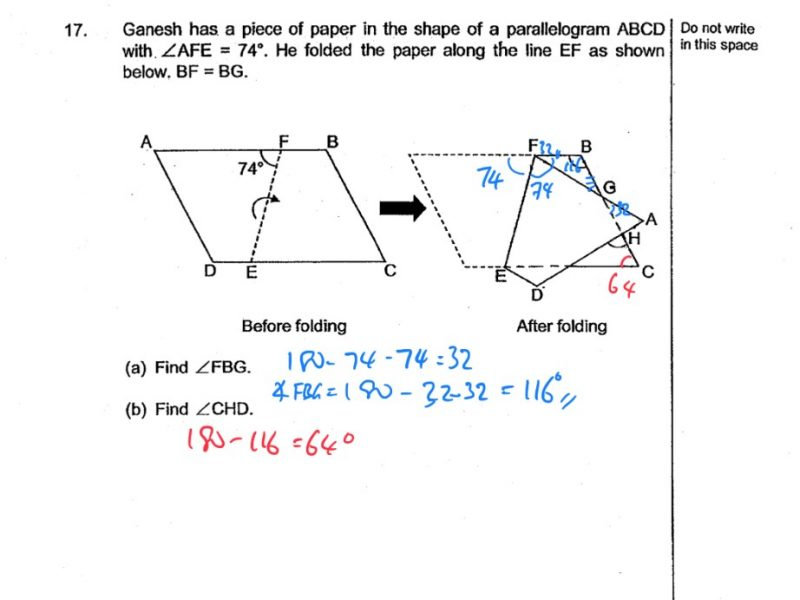# QuestionHi there, need help to solve part (b).

Source: Catholic High

From your working on the picture, you already have Angle FBG = 116, and Angle HCE = 64.

Continuing from there…

Angle GAH = Angle HCE = 64 (opp angles of a parallelogram)

Angle GHA = 180 – Angle GAH – Angle HGA = 180 – 64 – 32 = 84 (angles in a triangle)

Angle CHD = Angle GHA = 84 (vertically opp angles)